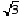Home Math Calculators Math  Flashcards Math Games Math  Homework Math Lessons Math Terms Math Worksheets Math Tools
You Are In Math Glossary of Terms

That is, a number which is having its square root taken (or cube root, 4th root, 5th root, nth root, etc.)

Example:

3 is the radicand inIn √x, "x" is the radicand.